Contents Articles Behaviors Books Director News Director Web Sites FAQ Games Mailing Lists News Groups Project Examples Reviews Software Tools Useful Web Sites Utilities Xtras

 Don't miss these#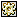Random numbers from file names -w/ BuddyAPI

 Compatibilities: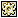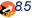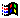Required Xtras: Buddy API This item has not yet been rated

Author: psykopath (website)

Gets random file names of the hard disk, gets a letter out of the file name,a nd creates a number from it. Use in the form rand(num, mode) Eg. rand(40, "float") would create floating point numbers up to 40 mode can be "float" or "integer" Put into a movie script to make available to all scripts.

 on rand lim, mode   --CREATES RANDOM NUMBERS FROM LETTERS IN FILE NAMES ON THE HARD DISK   tString = string(the ticks)   tCount = tString.char.count   tVar = integer( tString.char[tCount] )   tVar2 = integer( tString.char[tCount-1] )   case tVar2 of     1: --set 1 of numbers       case tVar of         0: cFile = 15         1: cFile = 19         2: cFile = 9         3: cFile = 11         4: cFile = 7         5: cFile = 13         6: cFile = 5         7: cFile = 3         8: cFile = 17         9: cFile = 1       end case     2: --set 2 of numbers       case tVar of         0: cFile = 11         1: cFile = 15         2: cFile = 17         3: cFile = 19         4: cFile = 13         5: cFile = 7         6: cFile = 3         7: cFile = 1         8: cFile = 9         9: cFile = 5       end case     3: --set 3 of numbers       case tVar of         0: cFile = 1         1: cFile = 5         2: cFile = 9         3: cFile = 13         4: cFile = 17         5: cFile = 19         6: cFile = 15         7: cFile = 11         8: cFile = 7         9: cFile = 3       end case     4: --set 4 of numbers       case tVar of         0: cFile = 3         1: cFile = 7         2: cFile = 11         3: cFile = 15         4: cFile = 19         5: cFile = 17         6: cFile = 13         7: cFile = 9         8: cFile = 5         9: cFile = 1       end case     5: --set 5 of numbers       case tVar of         0: cFile = 15         1: cFile = 11         2: cFile = 7         3: cFile = 3         4: cFile = 1         5: cFile = 5         6: cFile = 9         7: cFile = 13         8: cFile = 17         9: cFile = 19       end case     6: --set 6 of numbers       case tVar of         0: cFile = 7         1: cFile = 5         2: cFile = 9         3: cFile = 1         4: cFile = 13         5: cFile = 17         6: cFile = 15         7: cFile = 3         8: cFile = 19         9: cFile = 11       end case     7: --set 7 of numbers       case tVar of         0: cFile = 2         1: cFile = 4         2: cFile = 6         3: cFile = 8         4: cFile = 10         5: cFile = 12         6: cFile = 14         7: cFile = 16         8: cFile = 18         9: cFile = 20       end case     8: --set 8 of numbers       case tVar of         0: cFile = 20         1: cFile = 18         2: cFile = 16         3: cFile = 14         4: cFile = 12         5: cFile = 10         6: cFile = 8         7: cFile = 6         8: cFile = 4         9: cFile = 2       end case     9: --set 9 of numbers       case tVar of         0: cFile = 4         1: cFile = 14         2: cFile = 8         3: cFile = 18         4: cFile = 12         5: cFile = 10         6: cFile = 20         7: cFile = 6         8: cFile = 16         9: cFile = 2       end case     0: --set 10 of numbers       case tVar of         0: cFile = 1         1: cFile = 10         2: cFile = 2         3: cFile = 9         4: cFile = 3         5: cFile = 8         6: cFile = 4         7: cFile = 7         8: cFile = 5         9: cFile = 6       end case   end case   driveList = badisklist()   if driveList.getPos("c:") <> 0 then     if the platform contains "Windows" then       myPath = "C:"     else       myPath = driveList     end if   else     myPath = driveList   end if   myFile = EMPTY   repeat while myFile = EMPTY     myFile = getNthFileNameInFolder(myPath, cFile)     cFile = cFile-1   end repeat      tString = string(the milliseconds)   tCount = tString.char.count   tVar = integer( tString.char[tCount] )   case tVar of     0: cLetter= 1     1: cLetter = 6     2: cLetter = 2     3: cLetter = 7     4: cLetter = 3     5: cLetter = 8     6: cLetter = 4     7: cLetter = 9     8: cLetter = 5     9: cLetter = 10   end case   myChar = ""   repeat while myChar = ""     myChar = myFile.char[cLetter]     cLetter = cLetter-1   end repeat   myNum = chartonum(myChar)   fNum = ( (myNum-32.0)/90.0 )*lim   case mode of     "integer":       return integer(fNum)     "float":       return float(fNum)   end case end

 Contact MMI 36 South Court Sq Suite 300 Newnan, GA 30263 USA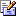Send e-mail